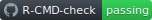This package provides means of estimating joint models for a mixture of different types of markers and different types of survival outcomes. The models are estimated with Gaussian variational approximations (GVAs). Description of the supported models and multiple examples are given in this document. A presentation of the package is available here which includes a simulation study to investigate bias of the parameter estimates and coverage of approximate likelihood ratio based confidence intervals.

A comparison with the JM, JMbayes, and joineRML is provided on Github at github.com/boennecd/VAJointSurv/tree/main/comparisons.

## Installation

The package can be installed from Github by calling:

remotes::install_github("boennecd/VAJointSurv", build_vignettes = TRUE)

## The Model

We will start by covering the model, then cover some examples, and end with details about the implementation.

### The Markers

We assume that there are $$L$$ observed markers at each time point. We let $$\vec Y_{ij}$$ denote the $$j$$th observed markers for individual $$i$$ and let $$s_{ij}$$ be the observation time. The model for the markers is

\begin{align*} \vec Y_{ij}&= \vec\mu_i(s_{ij}, \vec U_i) + \vec\epsilon_{ij} \\ \epsilon_{ij} &\sim N^{(L)}(\vec 0, \Sigma) \\ \mu_{i1}(s, \vec U_i) &= \vec x_{i1}^\top\vec\gamma_1 + \vec g_1(s)^\top\vec\beta_1 + \vec m_1(s)^\top\vec U_{i1} \\ \vdots &\hphantom{=}\vdots\\\ \mu_{iL}(s, \vec U_i) &= \vec x_{iL}^\top\vec\gamma_L + \vec g_L(s)^\top\vec\beta_L + \vec m_L(s)^\top\vec U_{iL} \\ \vec U_i &= \begin{pmatrix} \vec U_{i1} \\ \vdots \\ \vec U_{iL} \end{pmatrix}\sim N^{(R)}(\vec0, \Psi) \end{align*}

where $$\vec\epsilon_{ij}$$ is an error term of the observation, $$\vec U_i$$ is the $$R$$ dimensional unobserved random effect for individual $$i$$, and the $$\vec g_k$$s and $$\vec m_k$$s are basis expansions. We can write the model more compactly by letting

$G(s) = \begin{pmatrix} \vec g_1(s)^\top & 0^\top & \cdots & \vec 0^\top \\ \vec 0^\top & \vec g_2(s)^\top & \ddots & \vdots \\ \vdots & \ddots & \ddots & \vec 0^\top \\ \vec 0^\top & \cdots & \vec 0^\top & \vec g_L(s)^\top \end{pmatrix}$

and defining $$M(s)$$ and $$X_i$$ similarly. Furthermore, let $$\vec\gamma = (\vec\gamma_1^\top,\dots,\vec\gamma_L^\top)$$ and define $$\vec\beta$$ similarly. Then the conditional mean vector at time $$s_{ij}$$ is

$\vec\mu_i(s_{ij}, \vec U_i) = X_i\vec\gamma + G(s_{ij})\vec\beta + M(s_{ij})\vec U_i.$

### The Survival Outcomes

We assume that there are $$H$$ different types of survival outcomes. The conditional hazards of the survival outcomes are

\begin{align*} h_{i1}(t\mid \vec U_i, \vec\xi_i) &= \exp\left( \vec z_{i1}^\top\vec \delta_1 + \omega_1^\top \vec b_1(t) + \vec\alpha_1^\top M(t)\vec U_i + \xi_{i1} \right) \\ \vdots &\hphantom{=}\vdots \\ h_{iH}(t\mid \vec U_i,\vec \xi_i) &= \exp\left( \vec z_{iL}^\top\vec \delta_H + \omega_H^\top\vec b_H(t) + \vec\alpha_H^\top M(t)\vec U_i + \xi_{iH} \right) \\ \vec\xi_i = \begin{pmatrix}\xi_{i1} \\ \vdots \\ \xi_{iH}\end{pmatrix} &\sim N^{(H)}(\vec 0, \Xi). \end{align*}

The $$\vec\alpha$$s are association parameters which makes the markers and the survival outcomes marginally dependent. The $$\exp\xi_{ih}$$s are frailty effects which makes the survival outcomes marginally dependent even if $$\vec\alpha = \vec 0$$. By default, these are not included as it is assumed that events are often terminal in which case identifying the frailty may be hard and evidence of a frailty is both evidence of non-proportional hazards and heterogeneity.

The observation process, the $$s_{ij}$$s, can be modeled as one of $$H$$ different types of survival process. This can be done by adding each $$s_{ij}$$ as a left-truncated outcome of the given type of process except for $$s_{i1}$$ which is not left-truncated.

#### More General Survival Sub-model

The conditional hazards shown above only allows the hazard to be associated with the population deviation for the mean marker through the current value

$M(t)\vec U_i = \begin{pmatrix} \vec m_1(t)^\top\vec U_{i1} \\ \vdots \\ \vec m_L(t)^\top\vec U_{i1} \end{pmatrix}.$

However, the researcher may be interested in using the cumulative value, $$\int^t \vec m_k(s)^\top ds\vec U_{ik}$$, the derivative, $$\partial\vec m_k(t)^\top\vec U_{ik}/\partial t$$, or a combination of these and the current value. This is supported in the package through the ders argument of surv_term. As an example, if we let $$\vec d_k(t) = \int^t \vec m_k(s) ds$$, $$\vec r_k(t) = \partial\vec m_k(t)/\partial t$$, and $$L = 3$$ then it is possible to replace the $$\vec\alpha_1^\top M(t)\vec U_i$$ in the hazard with

$\vec\alpha_1^\top\begin{pmatrix} \vec d_1(t)^\top \vec U_{i1} \\ \vec m_1(t)^\top \vec U_{i1} \\ \vec m_2(t)^\top \vec U_{i2} \\ \vec d_3(t)^\top \vec U_{i3} \\ \vec r_3(t)^\top \vec U_{i3} \\ \end{pmatrix}$

That is, a cumulative and current value for the first marker, only the current value for the second marker, and the cumulative and derivative for the third marker.

### Model Support

The package can handle

• Mixed type of basis expansions in $$G$$, $$M$$, and $$b_1,\dots,b_H$$.
• A maximum $$L = 31$$ different markers, and an arbitrary number survival outcome types, $$H$$ (though, identification of parameters quickly becomes an issue).
• Only observing a subset of the $$L$$ markers at a given point in time $$s_{ij}$$ (i.e. some may be missing).
• Any number of observed markers and survival outcomes for each individual.
• Left-truncation and right-censoring.
• Time-varying effects for covariates (both fixed and random effects) and non-proportional hazard effects for covariates.

The following is not supported

• Interval-censoring and left-censoring are not supported although this is not a complicated extension.
• There cannot be multiple observed markers of the same type at each point $$s_{ij}$$ for each individual $$i$$. This is not too complicated to implement but not yet implemented.

## Examples

We show a few examples of using this package and illustrate its API in this section. The examples includes:

We start first with some general remarks.

### General Remarks

There is not intercept by default in the splines. Usually, you almost always want one in the both the random effect and fixed effect part. The intercept is included by default in formula (unless you do . ~ ... - 1 and there is not factor variable). Thus, you do not want an intercept in the time_fixef in both marker_term and surv_term. On the other hand, you do need yourself to include the intercept in the time_rng argument of marker_term. Thus, a typical call may look like

mark <- marker_term(
y ~ X1 + X2, # there is an intercept by default
# intercept is FALSE by default
time_fixef = poly_term(time, degree = 3),
# we need to add the intercept
time_rng = poly_term(time, degree = 1, intercept = TRUE))

s_term <- surv_term(
Surv(tstop, event) ~ X1, # there is an intercept by default
# intercept is FALSE by default
time_fixef = poly_term(tstop, degree = 1))

You may run into computational issues if you have a very flexible random effect model. This is quite typical with mixed models in a frequentist setting also with other estimation methods. One outcome of a too flexible random effect model is slow convergence or a NaN output because some of the scale matrices do not have full rank.

### Univariate Marker Example

We make a comparison below with one marker only with the lme4 package. We do this as we can check that

• We get almost the same (the lower bound can be equal to the log marginal likelihood in this case).
• The lower bound is less than or equal to the log marginal likelihood.

lme4 should be used in this case as the linear model is tractable and this is used by the lme4 package. The computation time comparison is not fair as we use four threads with the methods in this package.

# settings for the simulation
library(splines)
g_func <- function(x)
ns(x, knots = c(.5, 1.5), Boundary.knots = c(0, 2))
m_func <- function(x)
ns(x, knots = 1, Boundary.knots = c(0, 2), intercept = TRUE)

fixef_vary_marker <- c(1.4, -1.2, -2.1) # beta
fixef_marker <- c(-.5, 1) # gamma

# Psi
vcov_vary <- structure(
c(0.18, 0.05, -0.05, 0.05, 0.34, -0.25, -0.05, -0.25, 0.24),
.Dim = c(3L, 3L))
vcov_marker <- matrix(.6^2, 1) # Sigma

# plot the true mean curve along with 95% confidence pointwise quantiles
library(VAJointSurv)
par(mar = c(5, 5, 1, 1))
plot_marker(
time_fixef = ns_term(
knots = c(.5, 1.5), Boundary.knots = c(0, 2)),
time_rng = ns_term(
knots = 1, Boundary.knots = c(0, 2), intercept = TRUE),
fixef_vary = fixef_vary_marker, x_range = c(0, 2), vcov_vary = vcov_vary)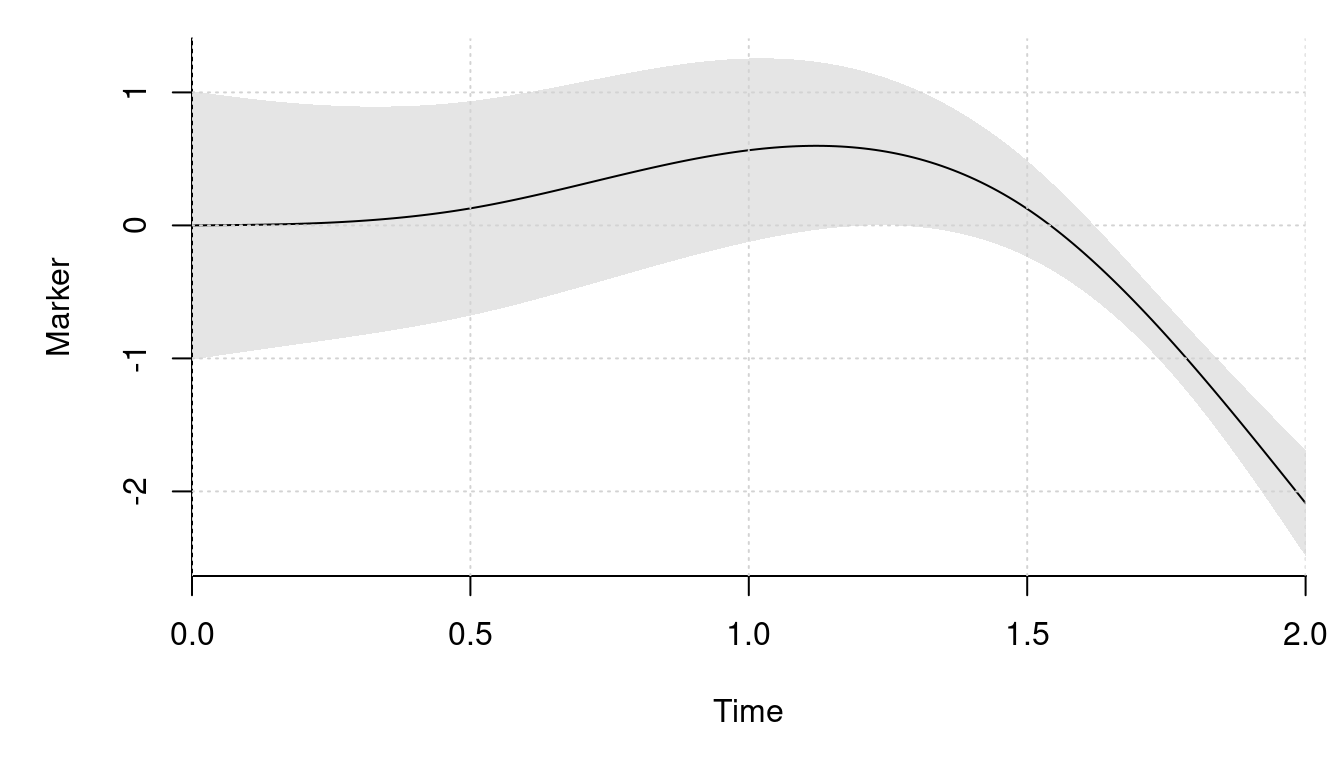library(mvtnorm)
# simulates from the model by sampling a given number of individuals
sim_dat <- function(n_ids){
# simulate the outcomes
sig_sqrt <- sqrt(vcov_marker)
dat <- lapply(1:n_ids, function(id){
# sample the number of outcomes and the fixed effect covariates
n_obs <- sample.int(8L, 1L)
obs_time <- sort(runif(n_obs, 0, 2))
X <- cbind(1, rnorm(n_obs))
colnames(X) <- paste0("X", 1:NCOL(X) - 1L)

# sample the outcomes
U <- drop(rmvnorm(1, sigma = vcov_vary))
eta <- X %*% fixef_marker + drop(g_func(obs_time)) %*% fixef_vary_marker +
drop(m_func(obs_time)) %*% U
y <- drop(eta) + rnorm(n_obs, sd = sig_sqrt)

cbind(y = y, X = X[, -1, drop = FALSE], time = obs_time, id = id)
})

# combine the data and return
out <- as.data.frame(do.call(rbind, dat))
out$id <- as.integer(out$id)
out[sample.int(NROW(out)), ] # the order does not matter
}

# sample a moderately large data set
set.seed(2)
dat <- sim_dat(2000L)

# the number of observed outcomes
nrow(dat)
#>  8992

# example of one individual's outcomes
subset(dat, id == 1)
#>         y       X1   time id
#> 5 -3.6206 -0.23970 1.8877  1
#> 2  0.1270 -0.08025 1.1467  1
#> 3 -0.3545  0.13242 1.4047  1
#> 1 -1.1089 -1.13038 0.3361  1
#> 4 -0.2127  0.70795 1.8869  1

# fit the model with lme4
library(lme4)
system.time(
fit <- lmer(y ~ X1 + g_func(time) + (m_func(time) - 1| id), dat,
control = lmerControl(optimizer = "bobyqa"),
# to compare with the lower bound from this package
REML = FALSE))
#>    user  system elapsed
#>   0.815   0.008   0.837

# the maximum log likelihood
print(logLik(fit), digits = 8)
#> 'log Lik.' -9161.7723 (df=12)

# estimate the model with this package. Get the object we need for the
# optimization
system.time(comp_obj <- joint_ms_ptr(
markers = marker_term(
y ~ X1, id = id, dat,
time_fixef = ns_term(time, knots = c(.5, 1.5), Boundary.knots = c(0, 2)),
time_rng = ns_term(time, knots = 1, Boundary.knots = c(0, 2),
intercept = TRUE)),
#>    user  system elapsed
#>   0.020   0.000   0.021

# get the starting values
system.time(start_val <- joint_ms_start_val(comp_obj))
#>    user  system elapsed
#>   2.241   0.012   0.948

# lower bound at the starting values
print(-attr(start_val, "value"), digits = 8)
#>  -9161.7781

# check that the gradient is correct
f <- function(x){
start_val[seq_along(x)] <- x
joint_ms_lb(comp_obj, start_val)
}

head(joint_ms_lb_gr(comp_obj, start_val), 12 + 2 * 9))
#>  "Mean relative difference: 1.029e-05"

# find the maximum lower bound estimate
system.time(opt_out <- joint_ms_opt(comp_obj, par = start_val, max_it = 1000L,
cg_tol = .2, c2 = .1))
#>    user  system elapsed
#>   0.505   0.000   0.128

# check the gradient norm. We may need to reduce the convergence tolerance if
# this is not small. In can also be a sign of convergence issues
sqrt(sum(joint_ms_lb_gr(comp_obj, opt_out$par)^2)) #>  0.7437 opt_out$info # convergence code (0 == 'OK')
#>  0
print(-opt_out$value, digits = 8) # maximum lower bound value #>  -9161.7762 print(logLik(fit), digits = 8) # maximum likelihood from lme4 #> 'log Lik.' -9161.7723 (df=12) # compare the estimates with the actual values. Start with the fixed effects fmt_ests <- joint_ms_format(comp_obj, opt_out$par)
rbind(lme4 = fixef(fit),
This package = do.call(c, fmt_ests$markers[]), Actual = c(fixef_marker, fixef_vary_marker)) #> (Intercept) X1 g_func(time)1 g_func(time)2 g_func(time)3 #> lme4 -0.5206 1.007 1.372 -1.104 -2.089 #> This package -0.5207 1.007 1.372 -1.104 -2.089 #> Actual -0.5000 1.000 1.400 -1.200 -2.100 # then the random effect covariance matrix vcov_est <- VarCorr(fit)[["id"]] attributes(vcov_est)[c("stddev", "correlation")] <- NULL vcov_est # lme4 #> m_func(time)1 m_func(time)2 m_func(time)3 #> m_func(time)1 0.16228 0.09634 -0.04534 #> m_func(time)2 0.09634 0.29559 -0.26821 #> m_func(time)3 -0.04534 -0.26821 0.29652 fmt_ests$vcov$vcov_vary # this package #> [,1] [,2] [,3] #> [1,] 0.16581 0.0955 -0.04641 #> [2,] 0.09550 0.2955 -0.26790 #> [3,] -0.04641 -0.2679 0.29643 vcov_vary # the actual values #> [,1] [,2] [,3] #> [1,] 0.18 0.05 -0.05 #> [2,] 0.05 0.34 -0.25 #> [3,] -0.05 -0.25 0.24 # the error term variance c(lme4 = attr(VarCorr(fit), "sc")^2, This package = fmt_ests$vcov$vcov_marker, Actual = vcov_marker) #> lme4 This package Actual #> 0.3667 0.3665 0.3600 #### Note on Basis Expansions This is a technical section which you may skip. Special basis expansions for e.g. poly and ns are implemented in R as poly_term and ns_term. The reason is that the integration shown in the Survival Outcomes section has to be done many times. Thus, we have implemented all the expansions in C++ to be used in C++ to reduce the cost of the evaluations. The _term functions provide the R interface to the C++ functions. Each return a list with elements like their R counterparts and an eval element. The latter element can be used to evaluate the basis, the derivatives of the basis if the der argument is greater than zero, or the integral $\vec d(u) = \int_l^u \vec m(s) ds$ if the der argument is equal to minus one. The lower limit is passed with the lower_limit argument to the eval function. We illustrate this below first with a raw polynomial. We also show that the derivatives and integral are correct. # raw polynomial with an without an intercept pl <- poly_term(degree = 3, raw = TRUE, intercept = TRUE) pl_no_inter <- poly_term(degree = 3, raw = TRUE, intercept = FALSE) t(pl$eval(1:2))
#>      [,1] [,2] [,3] [,4]
#> [1,]    1    1    1    1
#> [2,]    1    2    4    8
t(pl_no_inter$eval(1:2)) #> [,1] [,2] [,3] #> [1,] 1 1 1 #> [2,] 2 4 8 outer(1:2, 0:3, ^) # R version #> [,1] [,2] [,3] [,4] #> [1,] 1 1 1 1 #> [2,] 1 2 4 8 # derivatives library(numDeriv) t(pl$eval(2:3, der = 1))
#>      [,1] [,2] [,3] [,4]
#> [1,]    0    1    4   12
#> [2,]    0    1    6   27
t(pl_no_inter$eval(2:3, der = 1)) #> [,1] [,2] [,3] #> [1,] 1 4 12 #> [2,] 1 6 27 t(pl$eval(2:3, der = 2)) # second derivative
#>      [,1] [,2] [,3] [,4]
#> [1,]    0    0    2   12
#> [2,]    0    0    2   18
t(pl_no_inter$eval(2:3, der = 2)) # second derivative #> [,1] [,2] [,3] #> [1,] 0 2 12 #> [2,] 0 2 18 # trivial to do compute by hand but we do it with numDeriv anyway t(sapply(2:3, function(x) jacobian(function(z) outer(z, 0:3, ^), x))) #> [,1] [,2] [,3] [,4] #> [1,] 0 1 4 12 #> [2,] 0 1 6 27 # integral t(pl$eval(3:4, der = -1, lower_limit = 0))
#>      [,1] [,2]  [,3]  [,4]
#> [1,]    3  4.5  9.00 20.25
#> [2,]    4  8.0 21.33 64.00
t(pl_no_inter$eval(3:4, der = -1, lower_limit = 0)) #> [,1] [,2] [,3] #> [1,] 4.5 9.00 20.25 #> [2,] 8.0 21.33 64.00 # we could do this by hand but we write a function which we can reuse do_int <- function(xs, f, lower_limit){ n_terms <- length(f(xs)) g <- function(x, i) f(x)[, i] outer(xs, 1:n_terms, Vectorize( function(x, i) integrate(g, lower_limit, x, i = i)$value))
}
do_int(3:4, function(x) outer(x, 0:3, ^), 0)
#>      [,1] [,2]  [,3]  [,4]
#> [1,]    3  4.5  9.00 20.25
#> [2,]    4  8.0 21.33 64.00

The functionality also works for orthogonal polynomials.

# equally works with orthogonal polynomials
xx <- seq(0, 4, length.out = 10)
pl <- poly_term(xx, degree = 3, raw = FALSE, intercept = TRUE)
t(pl$eval(2:3)) #> [,1] [,2] [,3] [,4] #> [1,] 1 5.500e-17 -0.3590 2.391e-16 #> [2,] 1 2.477e-01 -0.1387 -3.881e-01 predict(poly(xx, degree = 3), 2:3) #> 1 2 3 #> [1,] 5.500e-17 -0.3590 2.391e-16 #> [2,] 2.477e-01 -0.1387 -3.881e-01 # derivatives t(pl$eval(2:3, der = 1))
#>      [,1]   [,2]      [,3]     [,4]
#> [1,]    0 0.2477 1.110e-16 -0.59310
#> [2,]    0 0.2477 4.406e-01  0.02176
t(sapply(2:3, function(x)
jacobian(function(z) predict(poly(xx, degree = 3), z), x)))
#>        [,1]   [,2]     [,3]
#> [1,] 0.2477 0.0000 -0.59310
#> [2,] 0.2477 0.4406  0.02176

# integral
t(pl$eval(3:4, der = -1, lower_limit = 0)) #> [,1] [,2] [,3] [,4] #> [1,] 3 -3.716e-01 -0.4162 1.211e-01 #> [2,] 4 2.220e-16 -0.2611 1.776e-15 do_int(3:4, function(x) predict(poly(xx, degree = 3), x), 0) #> [,1] [,2] [,3] #> [1,] -3.716e-01 -0.4162 1.211e-01 #> [2,] 2.248e-16 -0.2611 5.231e-16 The derivatives and integral are also available for B-splines and natural cubic splines through the ns_term and bs_term functions. # B-splines library(splines) xx <- 0:10 b <- bs_term(xx, df = 4, Boundary.knots = range(xx)) t(b$eval(4.33))
#>        [,1]   [,2]   [,3] [,4]
#> [1,] 0.3598 0.4755 0.1624    0
c(predict(bs(xx, df = 4, Boundary.knots = range(xx)), 4.33))
#>  0.3598 0.4755 0.1624 0.0000

# derivatives
t(b$eval(4.33, der = 1)) #> [,1] [,2] [,3] [,4] #> [1,] -0.1713 0.06963 0.1125 0 f <- function(z) suppressWarnings( predict(bs(xx, df = 4, Boundary.knots = range(xx)), z)) t(jacobian(f, 4.33)) #> [,1] [,2] [,3] [,4] #> [1,] -0.1713 0.06963 0.1125 0 # integrals all.equal( t(b$eval(12, der = -1, lower_limit = 11)), do_int(12, f, 11), 1e-6)
#>  TRUE
all.equal( t(b$eval(11, der = -1, lower_limit = 0)), do_int(11, f, 0), 1e-6) #>  TRUE all.equal(-t(b$eval( 0, der = -1, lower_limit = 11)), do_int(11, f,  0), 1e-6)
#>  TRUE
all.equal( t(b$eval( 5, der = -1, lower_limit = 1)), do_int( 5, f, 1), 1e-6) #>  TRUE all.equal( t(b$eval(-1, der = -1, lower_limit =  2)), do_int(-1, f,  2), 1e-6)
#>  TRUE

# natural cubic spline
xx <- 0:10
n <- ns_term(xx, df = 3, Boundary.knots = range(xx))
t(n$eval(4.33)) #> [,1] [,2] [,3] #> [1,] 0.1723 0.5256 -0.346 c(predict(ns(xx, df = 3, Boundary.knots = range(xx)), 4.33)) #>  0.1723 0.5256 -0.3460 # derivatives t(n$eval(4.33, der = 1))
#>        [,1]     [,2]    [,3]
#> [1,] 0.2186 -0.05737 0.05165
f <- function(z) predict(ns(xx, df = 3, Boundary.knots = range(xx)), z)
t(jacobian(f, 4.33))
#>        [,1]     [,2]    [,3]
#> [1,] 0.2186 -0.05737 0.05165

# integrals
all.equal( t(n$eval(12, der = -1, lower_limit = 11)), do_int(12, f, 11), 1e-6) #>  TRUE all.equal( t(n$eval(11, der = -1, lower_limit =  0)), do_int(11, f,  0), 1e-6)
#>  TRUE
all.equal(-t(n$eval( 0, der = -1, lower_limit = 11)), do_int(11, f, 0), 1e-6) #>  TRUE all.equal( t(n$eval( 7, der = -1, lower_limit = -2)), do_int( 7, f, -2), 1e-6)
#>  TRUE
all.equal( t(n$eval(-1, der = -1, lower_limit = -3)), do_int(-1, f, -3), 1e-6) #>  TRUE Caution: the B-splines are only tested with degree = 3! #### Log Transformations The expansions can also be used on the log scale. This is mainly implemented to allow the log of the baseline hazard to be parameterized in terms of an expansions in log time to have the Weibull model as a special case. A few examples are given below. # raw log polynomial with an without an intercept pl <- poly_term(degree = 3, raw = TRUE, intercept = TRUE, use_log = TRUE) pl_no_inter <- poly_term(degree = 3, raw = TRUE, intercept = FALSE, use_log = TRUE) t(pl$eval(1:2))
#>      [,1]   [,2]   [,3]  [,4]
#> [1,]    1 0.0000 0.0000 0.000
#> [2,]    1 0.6931 0.4805 0.333
t(pl_no_inter$eval(1:2)) #> [,1] [,2] [,3] #> [1,] 0.0000 0.0000 0.000 #> [2,] 0.6931 0.4805 0.333 outer(log(1:2), 0:3, ^) # R version #> [,1] [,2] [,3] [,4] #> [1,] 1 0.0000 0.0000 0.000 #> [2,] 1 0.6931 0.4805 0.333 # derivatives library(numDeriv) t(pl$eval(2:3, der = 1))
#>      [,1]   [,2]   [,3]   [,4]
#> [1,]    0 0.5000 0.6931 0.7207
#> [2,]    0 0.3333 0.7324 1.2069
t(pl_no_inter$eval(2:3, der = 1)) #> [,1] [,2] [,3] #> [1,] 0.5000 0.6931 0.7207 #> [2,] 0.3333 0.7324 1.2069 # trivial to do compute by hand but we do it with numDeriv anyway t(sapply(2:3, function(x) jacobian(function(z) outer(log(z), 0:3, ^), x))) #> [,1] [,2] [,3] [,4] #> [1,] 0 0.5000 0.6931 0.7207 #> [2,] 0 0.3333 0.7324 1.2069 # B-splines library(splines) xx <- 1:10 b <- bs_term(xx, df = 4, use_log = TRUE) t(b$eval(4.33))
#>        [,1]   [,2]   [,3] [,4]
#> [1,] 0.1736 0.4746 0.3491    0
c(predict(bs(log(xx), df = 4), log(4.33)))
#>  0.1736 0.4746 0.3491 0.0000

# derivatives
t(b$eval(4.33, der = 1)) #> [,1] [,2] [,3] [,4] #> [1,] -0.1223 -0.03495 0.165 0 f <- function(z) suppressWarnings(predict(bs(log(xx), df = 4), log(z))) t(jacobian(f, 4.33)) #> [,1] [,2] [,3] [,4] #> [1,] -0.1223 -0.03495 0.165 0 # natural cubic spline xx <- 1:10 n <- ns_term(xx, df = 3, use_log = TRUE) t(n$eval(4.33))
#>        [,1]   [,2]   [,3]
#> [1,] 0.3855 0.4276 -0.307
c(predict(ns(log(xx), df = 3), log(4.33)))
#>   0.3855  0.4276 -0.3070

# derivatives
t(n$eval(4.33, der = 1)) #> [,1] [,2] [,3] #> [1,] 0.2536 -0.09559 0.07548 f <- function(z) predict(ns(log(xx), df = 3), log(z)) t(jacobian(f, 4.33)) #> [,1] [,2] [,3] #> [1,] 0.2536 -0.09559 0.07548 #### Time-varying Effects We may also be interested in • Time-varying fixed effects for a covariate. • Time-varying random effects for a covariate. • Non-proportional hazard effects for a covariate. The way that we allow for this is with the weighted_term and stacked_term functions. The weighted_term function takes a basis expansion which we can denote as $$\vec g(t)$$ and a symbol (weight) for a covariate which we denote by $$x$$ and computes $$x\vec g(t)$$. Two examples of using the function are given below. Note that the symbol is not evaluated till you call the eval function on the returned object. # define two bases term_bmi <- weighted_term( poly_term(degree = 2, intercept = TRUE, raw = TRUE), weight = bmi) term_sex <- weighted_term( poly_term(degree = 1, intercept = TRUE, raw = TRUE), weight = is_male) # use the two bases in an example ti <- 2:3 df <- data.frame(bmi = c(23, 25), is_male = c(0, 1)) term_bmi$eval(x = ti, newdata = df)
#>      [,1] [,2]
#> [1,]   23   25
#> [2,]   46   75
#> [3,]   92  225
term_sex$eval(x = ti, newdata = df) #> [,1] [,2] #> [1,] 0 1 #> [2,] 0 3 The stacked_term function takes a number of expansions and stack them on top of each other. This illustrated below. # add an "intercept" to the two other terms combined term_comb <- stacked_term(poly_term(degree = 2, intercept = TRUE, raw = TRUE), term_bmi, term_sex) term_comb$eval(ti, newdata = df)
#>      [,1] [,2]
#> [1,]    1    1
#> [2,]    2    3
#> [3,]    4    9
#> [4,]   23   25
#> [5,]   46   75
#> [6,]   92  225
#> [7,]    0    1
#> [8,]    0    3

# it is a bit obscure but we can also weight the combined term
term_comb_sex <- weighted_term(term_comb, is_male)
term_comb_sex$eval(ti, newdata = df) #> [,1] [,2] #> [1,] 0 1 #> [2,] 0 3 #> [3,] 0 9 #> [4,] 0 25 #> [5,] 0 75 #> [6,] 0 225 #> [7,] 0 1 #> [8,] 0 3 ### Two Markers We provide an example with two markers instead of one. We observe one of the two markers or both at each observation time. There is also a closed form solution in this case. Thus, this is not the optimal way of doing this and it is only shown as an example and as a sanity check. # settings for the simulation library(splines) g_funcs <- list( function(x) ns(x, knots = c(.5, 1.5), Boundary.knots = c(0, 2)), function(x) # a raw polynomial outer(x, 1:2, ^)) m_funcs <- list( function(x) ns(x, knots = numeric(), Boundary.knots = c(0, 2), intercept = TRUE), function(x) # a raw polynomial outer(x, 0:1, ^)) fixef_vary_marker <- list(c(1.4, -1.2, -2.1), c(.5, .67)) # beta fixef_marker <- list(c(-.5, 1), .25) # gamma # Psi vcov_vary <- structure( c(0.35, 0.02, -0.05, 0.01, 0.02, 0.12, -0.06, -0.01, -0.05, -0.06, 0.32, 0.09, 0.01, -0.01, 0.09, 0.12), .Dim = c(4L, 4L)) vcov_marker <- matrix(c(.6^2, .1, .1, .4^2), 2) # Sigma # plot the markers' mean curve library(VAJointSurv) par(mar = c(5, 5, 1, 1)) plot_marker( time_fixef = ns_term( knots = c(.5, 1.5), Boundary.knots = c(0, 2)), time_rng = ns_term( knots = numeric(), Boundary.knots = c(0, 2), intercept = TRUE), fixef_vary = fixef_vary_marker[], x_range = c(0, 2), vcov_vary = vcov_vary[1:2, 1:2], ylab = "Marker 1")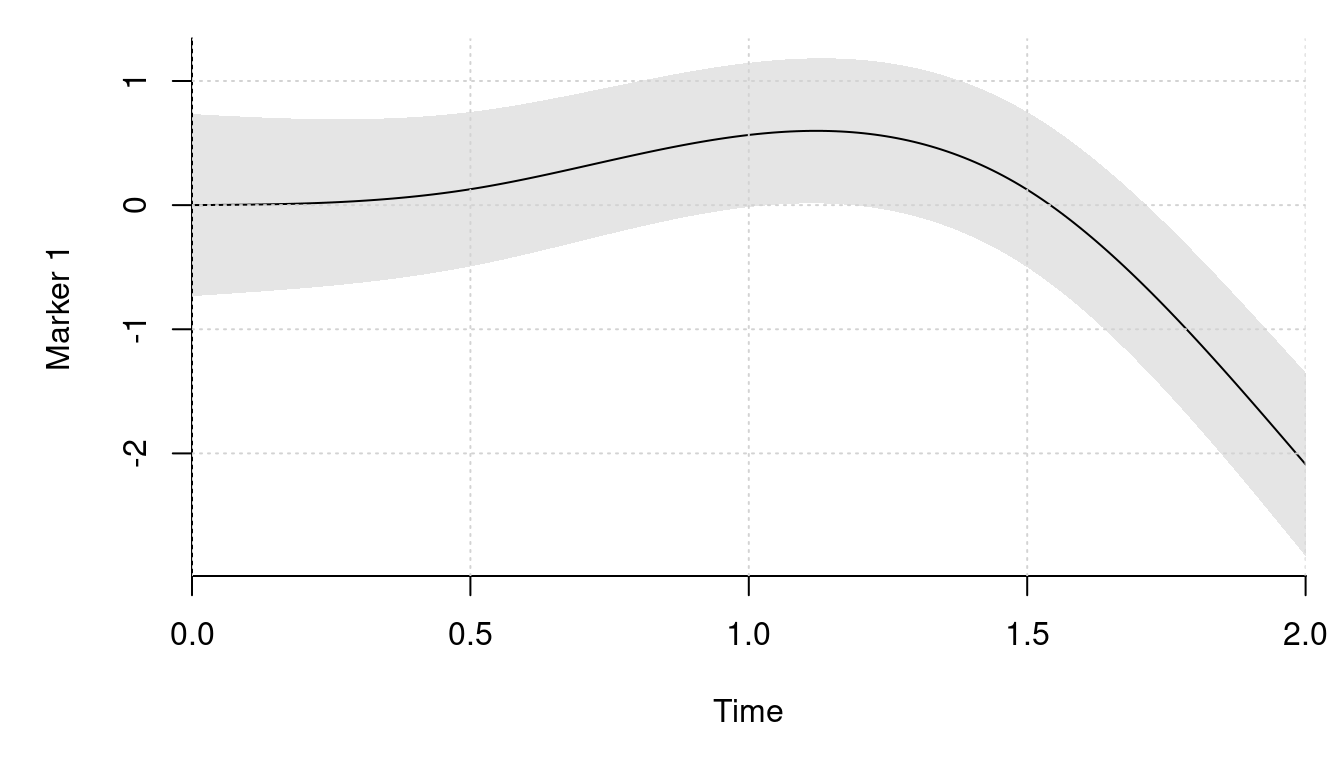plot_marker( time_fixef = poly_term(degree = 2, raw = TRUE), time_rng = poly_term(degree = 1, raw = TRUE, intercept = TRUE), fixef_vary = fixef_vary_marker[], x_range = c(0, 2), vcov_vary = vcov_vary[3:4, 3:4], ylab = "Marker 2")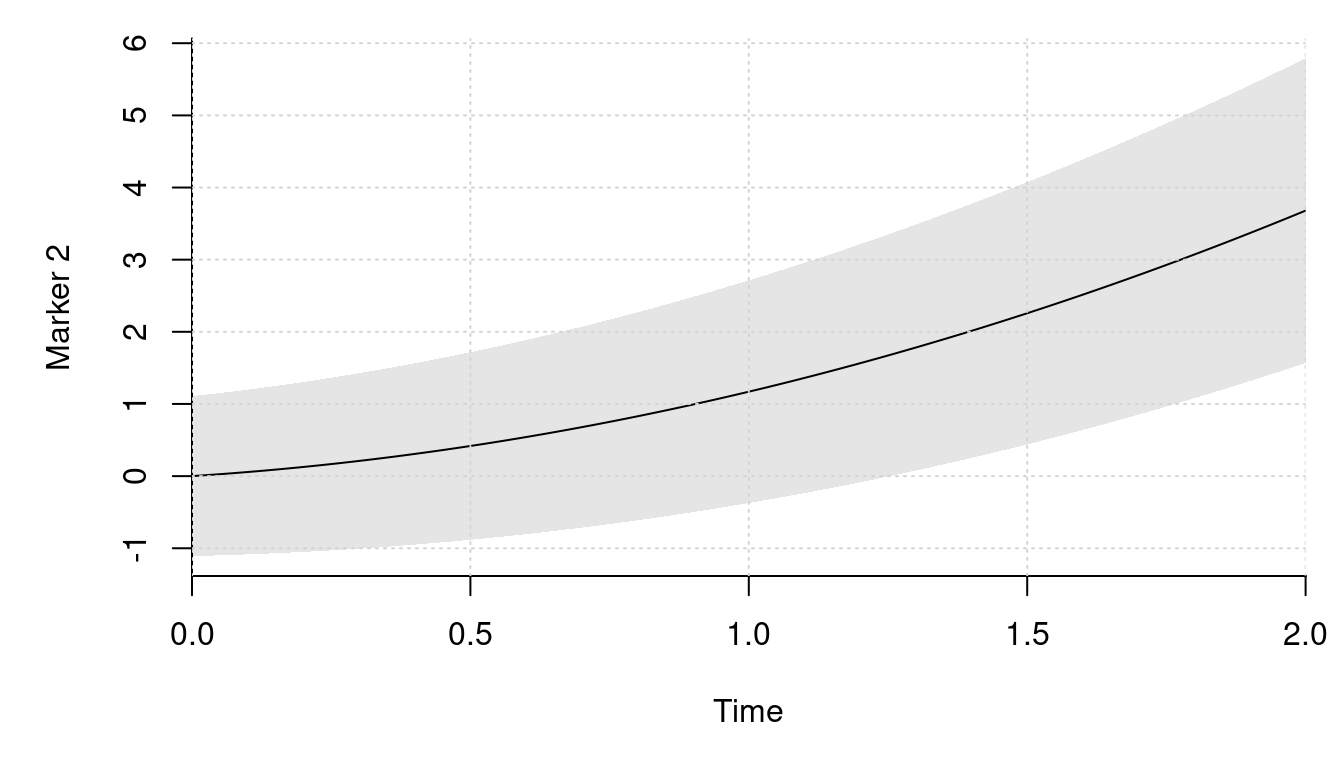library(mvtnorm) # simulates from the model by sampling a given number of individuals sim_dat <- function(n_ids){ # simulate the outcomes dat <- lapply(1:n_ids, function(id){ # sample the number of outcomes and the fixed effect covariates n_obs <- sample.int(8L, 1L) obs_time <- sort(runif(n_obs, 0, 2)) X1 <- cbind(1, rnorm(n_obs)) X2 <- matrix(1, n_obs) colnames(X1) <- paste0("X1_", 1:NCOL(X1) - 1L) colnames(X2) <- paste0("X2_", 1:NCOL(X2) - 1L) X <- list(X1, X2) # sample the outcomes U <- drop(rmvnorm(1, sigma = vcov_vary)) eta <- sapply(1:2, function(i) X[[i]] %*% fixef_marker[[i]] + drop(g_funcs[[i]](obs_time)) %*% fixef_vary_marker[[i]] + drop(m_funcs[[i]](obs_time)) %*% U[1:2 + (i == 2) * 2]) ys <- eta + rmvnorm(n_obs, sigma = vcov_marker) colnames(ys) <- paste0("Y", 1:2) # mask some observations do_mask <- sample.int(3L, n_obs, replace = TRUE) ys[do_mask == 2, 1] <- NA ys[do_mask == 3, 2] <- NA X <- do.call(cbind, lapply(X, function(x) x[, -1, drop = FALSE])) cbind(ys, X, time = obs_time, id = id) }) # combine the data and return out <- as.data.frame(do.call(rbind, dat)) out$id <- as.integer(out$id) out[sample.int(NROW(out)), ] # the order does not matter } # sample a moderately large data set set.seed(2) dat <- sim_dat(1000L) # example of the data for one individual subset(dat, id == 1) #> Y1 Y2 X1_1 time id #> 3 NA 3.1915 0.13242 1.4047 1 #> 5 NA 4.3373 -0.23970 1.8877 1 #> 4 NA 4.8558 0.70795 1.8869 1 #> 2 1.399 1.7030 -0.08025 1.1467 1 #> 1 -1.286 0.4379 -1.13038 0.3361 1 # estimate the model with this package. Get the object we need for the # optimization marker_1 <- marker_term( Y1 ~ X1_1, id = id, subset(dat, !is.na(Y1)), time_fixef = ns_term(time, knots = c(.5, 1.5), Boundary.knots = c(0, 2)), time_rng = ns_term(time, knots = numeric(), Boundary.knots = c(0, 2), intercept = TRUE)) marker_2 <- marker_term( Y2 ~ 1, id = id, subset(dat, !is.na(Y2)), time_fixef = poly_term(time, degree = 2, raw = TRUE), time_rng = poly_term(time, degree = 1, raw = TRUE, intercept = TRUE)) comp_obj <- joint_ms_ptr(markers = list(marker_1, marker_2), max_threads = 4L) rm(marker_1, marker_2) # get the starting values system.time(start_val <- joint_ms_start_val(comp_obj)) #> user system elapsed #> 2.086 0.004 0.615 # lower bound at the starting values print(-attr(start_val, "value"), digits = 8) #>  -5729.7904 # check that the gradient is correct f <- function(x){ start_val[seq_along(x)] <- x joint_ms_lb(comp_obj, start_val) } all.equal(numDeriv::grad(f, head(start_val, 22 + 2 * 14)), head(joint_ms_lb_gr(comp_obj, start_val), 22 + 2 * 14)) #>  "Mean relative difference: 0.0001464" # find the maximum lower bound estimate system.time(opt_out <- joint_ms_opt(comp_obj, par = start_val, max_it = 1000L, pre_method = 3L, cg_tol = .2, c2 = .1, gr_tol = .1)) #> user system elapsed #> 0.342 0.000 0.086 # we set gr_tol in the call so this is the convergence criterion for the # gradient sqrt(sum(joint_ms_lb_gr(comp_obj, opt_out$par)^2))
#>  0.09769
opt_out$info # convergence code (0 == 'OK') #>  0 print(-opt_out$value, digits = 8) # maximum lower bound value
#>  -5729.7904
opt_out$counts #> function gradient n_cg #> 42 30 41 # find the maximum lower bound with lbfgs library(lbfgsb3c) system.time(lbfgs_res <- lbfgsb3c( start_val, function(x) joint_ms_lb(comp_obj, x), function(x) joint_ms_lb_gr(comp_obj, x), control = list(factr = 1e-8, maxit = 10000L))) #> user system elapsed #> 36.863 0.016 9.284 lbfgs_res$convergence # convergence code (0 == 'OK')
#>  0
print(-lbfgs_res$value, digits = 8) # maximum lower bound value #>  -5729.7903 lbfgs_res$counts # may have hit maxit!
#>  4108 4108

# compare the estimates with the actual values. Start with the fixed effects
fmt_ests <- joint_ms_format(comp_obj, opt_out$par) fmt_ests_lbfgs <- joint_ms_format(comp_obj, lbfgs_res$par)

# the parameters for the first marker
fmt_ests$markers[] #>$fixef
#>  -0.4967  1.0249
#>
#> $fixef_vary #>  1.544 -1.237 -2.114 fmt_ests_lbfgs$markers[] # with lbfgs
#> $fixef #>  -0.4966 1.0249 #> #>$fixef_vary
#>   1.544 -1.238 -2.114

fixef_marker[] # true values
#>  -0.5  1.0
fixef_vary_marker[] # true values
#>   1.4 -1.2 -2.1

# the parameters for the second marker
fmt_ests$markers[] #>$fixef
#>  0.2552
#>
#> $fixef_vary #>  0.5381 0.6592 fmt_ests_lbfgs$markers[] # with lbfgs
#> $fixef #>  0.2552 #> #>$fixef_vary
#>  0.5382 0.6591
fixef_marker[] # true values
#>  0.25
fixef_vary_marker[] # true values
#>  0.50 0.67

# the parameters for covariance matrix of the random effects
fmt_ests$vcov$vcov_vary
#>            [,1]     [,2]      [,3]      [,4]
#> [1,]  0.4155040  0.02347 -0.006174 0.0004588
#> [2,]  0.0234666  0.19181 -0.087433 0.0167084
#> [3,] -0.0061743 -0.08743  0.331594 0.0688998
#> [4,]  0.0004588  0.01671  0.068900 0.0948096
fmt_ests_lbfgs$vcov$vcov_vary # with lbfgs
#>            [,1]     [,2]      [,3]      [,4]
#> [1,]  0.4155511  0.02345 -0.006134 0.0004161
#> [2,]  0.0234507  0.19154 -0.087515 0.0167512
#> [3,] -0.0061338 -0.08752  0.331641 0.0688570
#> [4,]  0.0004161  0.01675  0.068857 0.0948671
vcov_vary # the true values
#>       [,1]  [,2]  [,3]  [,4]
#> [1,]  0.35  0.02 -0.05  0.01
#> [2,]  0.02  0.12 -0.06 -0.01
#> [3,] -0.05 -0.06  0.32  0.09
#> [4,]  0.01 -0.01  0.09  0.12

# the parameters for the error term variance
fmt_ests$vcov$vcov_marker
#>        [,1]   [,2]
#> [1,] 0.3575 0.1003
#> [2,] 0.1003 0.1601
fmt_ests_lbfgs$vcov$vcov_marker # with lbfgs
#>        [,1]   [,2]
#> [1,] 0.3575 0.1003
#> [2,] 0.1003 0.1601
vcov_marker
#>      [,1] [,2]
#> [1,] 0.36 0.10
#> [2,] 0.10 0.16

### Recurrent Event

We simulate from a model with a recurrent event in this section. This is a more relevant example as there is not a closed form solution of the marginal likelihood.

# the survival parameters
fixef_surv <- c(-.5, .25) # delta
fixef_vary_surv <- c(.5, .1, -.2, .11) # omega
vcov_surv <- matrix(.2^2, 1) # Xi

library(splines)
b_func <- function(x)
bs(x, knots = 1, Boundary.knots = c(0, 2))

# sample a few survival curves and plot them
library(SimSurvNMarker)
# time points where we evaluate the conditional survival functions
tis <- seq(0, 2, length.out = 50)
set.seed(1)
# compute the conditional survival functions disregarding the fixed effect
surv_vals <- replicate(100, {
err <- rnorm(1, sd = sqrt(vcov_surv))
eval_surv_base_fun(
ti = tis, omega = fixef_vary_surv, b_func = b_func,
gl_dat = get_gl_rule(100), delta = fixef_surv + err)
})

par(mar = c(5, 5, 1, 1))
matplot(tis, surv_vals, type = "l", col = gray(0, .1), bty = "l", lty = 1,
ylim = c(0, 1), xaxs = "i", yaxs = "i", xlab = "Time",
ylab = "Conditional survival function")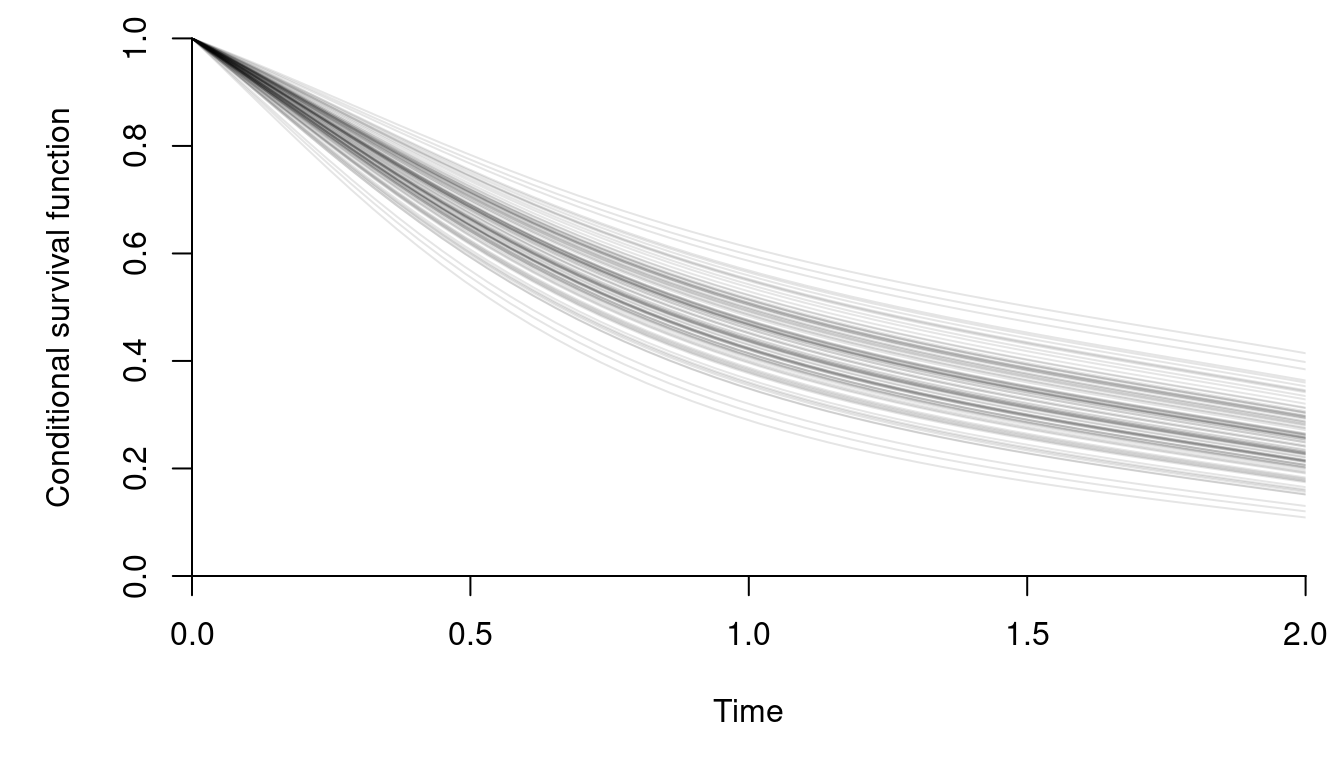# compute the average to get an estimate of the marginal survival curve
plot(tis, rowMeans(surv_vals), type = "l",  bty = "l", ylim = c(0, 1),
xaxs = "i", yaxs = "i", xlab = "Time",
ylab = "Marginal survival function")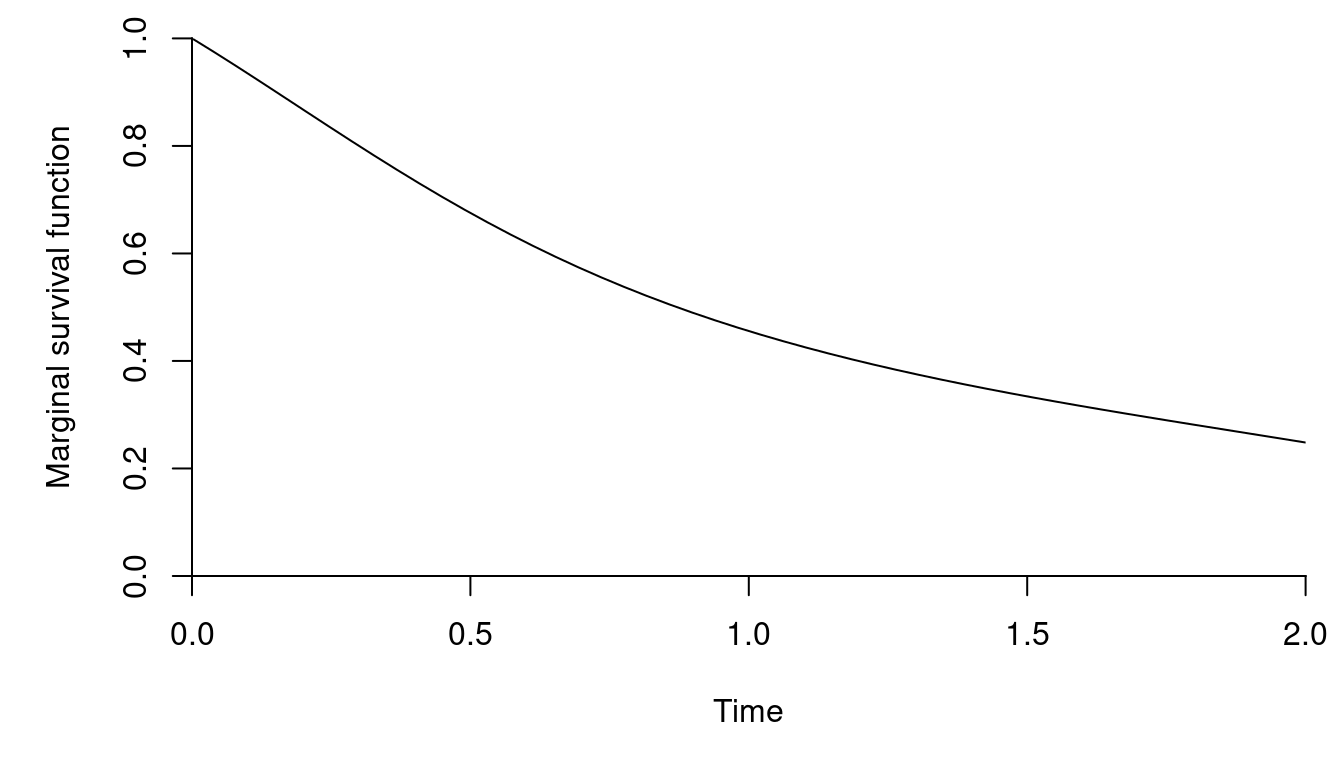# simulates from the model by sampling a given number of individuals
sim_dat <- function(n_ids){
# simulate the outcomes
gl_dat <- get_gl_rule(100L)
dat <- lapply(1:n_ids, function(id){
# simulate the survival outcome
rng_surv <- rnorm(1, sd = sqrt(vcov_surv))
Z <- c(1, runif(1, -1, 1))
log_haz_offset <- sum(Z * fixef_surv) + rng_surv

# the conditional survival function
surv_func <- function(ti)
eval_surv_base_fun(
ti = ti, omega = fixef_vary_surv, b_func = b_func, gl_dat = gl_dat,
delta = log_haz_offset)

# simulate the recurrent events one at a time
max_sample <- 10L
Z <- matrix(rep(Z, each = max_sample), max_sample)
event <- y <- lf_trunc <- rep(NA_real_, max_sample)
lf_trunc_i <- 0
rngs <- runif(max_sample)
left_trunc_surv <- 1
for(i in 1:max_sample){
# sample a random uniform variable and invert the survival function
rng_i <- rngs[i]
lf_trunc[i] <- lf_trunc_i

root_func <- function(x) rng_i - surv_func(x) / left_trunc_surv
if(root_func(2) < 0){
# the observation is right-censored and we can exit
y[i] <- 2
event[i] <- 0
break
}

# we need to invert the survival function to find the observation time
root <- uniroot(root_func, c(lf_trunc_i, 2), tol = 1e-6)
lf_trunc_i <- y[i] <- root$root event[i] <- 1 left_trunc_surv <- surv_func(y[i]) } # keep the needed data and return colnames(Z) <- paste0("Z_", 1:NCOL(Z) - 1L) surv_data <- cbind(lf_trunc = lf_trunc[1:i], y = y[1:i], event = event[1:i], Z[1:i, -1, drop = FALSE], id = id) list(surv_data = surv_data) }) # combine the data and return surv_data <- as.data.frame(do.call( rbind, lapply(dat, [[, "surv_data"))) surv_data$id <- as.integer(surv_data$id) # the order does not matter surv_data <- surv_data[sample.int(NROW(surv_data)), ] list(surv_data = surv_data) } # sample a moderately large data set set.seed(3) dat <- sim_dat(1000L) # we show a few properties of the data below NROW(dat$surv_data) # number of survival outcomes
#>  2388
sum(dat$surv_data$event) # number of observed events
#>  1388

# example of the data for three individuals
subset(dat$surv_data, id %in% 1:3) #> lf_trunc y event Z_1 id #> 5 1.6383 2.0000 0 0.1208 3 #> 3 0.0000 0.3526 1 0.1208 3 #> 4 0.3526 1.6383 1 0.1208 3 #> 1 0.0000 2.0000 0 -0.2301 1 #> 2 0.0000 2.0000 0 0.6594 2 # distribution of observed events per individual proportions(table(table(dat$surv_data$id) - 1L)) #> #> 0 1 2 3 4 5 6 #> 0.266 0.343 0.220 0.103 0.050 0.012 0.006 # estimate the model with this package. Get the object we need for the # optimization library(survival) library(VAJointSurv) surv_obj <- surv_term( Surv(lf_trunc, y, event) ~ Z_1, id = id, dat$surv_data,
time_fixef = bs_term(y, knots = 1, Boundary.knots = c(0, 2)),
with_frailty = TRUE)

comp_obj <- joint_ms_ptr(markers = list(),
survival_terms = surv_obj, max_threads = 4L)
rm(surv_obj)

# get the starting values
system.time(start_val <- joint_ms_start_val(comp_obj))
#>    user  system elapsed
#>   0.244   0.000   0.160

# lower bound at the starting values
print(-attr(start_val, "value"), digits = 8)
#>  -1864.1515

# check that the gradient is correct
f <- function(x){
start_val <- comp_obj$start_val start_val[seq_along(x)] <- x joint_ms_lb(comp_obj, start_val) } all.equal(numDeriv::grad(f, head(comp_obj$start_val, 7 + 2 * 2)),
head(joint_ms_lb_gr(comp_obj, comp_obj$start_val), 7 + 2 * 2), tolerance = 1e-6) #>  TRUE # find the maximum lower bound estimate system.time(opt_out <- joint_ms_opt(comp_obj, par = start_val, max_it = 1000L, pre_method = 3L, cg_tol = .2, c2 = .1)) #> user system elapsed #> 0.813 0.000 0.206 # check the gradient norm. We may need to reduce the convergence tolerance if # this is not small. In can also be a sign of convergence issues sqrt(sum(joint_ms_lb_gr(comp_obj, opt_out$par)^2))
#>  0.1184
opt_out$info # convergence code (0 == 'OK') #>  0 print(-opt_out$value, digits = 8) # maximum lower bound value
#>  -1863.2795
opt_out$counts #> function gradient n_cg #> 156 101 165 # check the estimates fmt_est <- joint_ms_format(comp_obj, opt_out$par)

rbind(estimate = fmt_est$survival[]$fixef, truth = fixef_surv)
#>
#> estimate -0.5058 0.2582
#> truth    -0.5000 0.2500
rbind(estimate = fmt_est$survival[]$fixef_vary, truth = fixef_vary_surv)
#>
#> estimate 0.3965 0.2539 -0.3217 0.07835
#> truth    0.5000 0.1000 -0.2000 0.11000
c(estimate = sqrt(fmt_est$vcov$vcov_surv), truth = sqrt(vcov_surv))
#> estimate    truth
#>    0.228    0.200

A quadrature rule is used to integrate the expected cumulative hazard. The user can pass any quadrature rule with $$n$$ nodes and weights each denoted by $$(n_i, w_i)$$ such that

$\int_0^1f(x) dx \approx \sum_{i = 1}^nw_i f(n_i).$

By default Gauss–Legendre quadrature is used. A very simple alternative is to use the midpoint rule. This is illustrated below.

# computes the midpoint rule
mid_rule <- function(n)
list(node = seq(0, 1, length.out = n), weight = rep(1 / n, n))

# compare the midpoint with different number of nodes
for(n in 2^(3:12)){
res <- joint_ms_lb(comp_obj, opt_out$par, n_threads = 4L, quad_rule = mid_rule(n)) cat(sprintf("# nodes, lower bound %5d %12.4f\n", n, res)) } #> # nodes, lower bound 8 1859.8265 #> # nodes, lower bound 16 1861.5170 #> # nodes, lower bound 32 1862.3858 #> # nodes, lower bound 64 1862.8294 #> # nodes, lower bound 128 1863.0536 #> # nodes, lower bound 256 1863.1663 #> # nodes, lower bound 512 1863.2228 #> # nodes, lower bound 1024 1863.2511 #> # nodes, lower bound 2048 1863.2652 #> # nodes, lower bound 4096 1863.2723 # the maximum lower bound value we got with Gauss–Legendre quadrature print(-opt_out$value, digits = 8)
#>  -1863.2795

We can compare this with using Gauss–Legendre quadrature with different number of nodes.

# get the rules
rules <- legendre.quadrature.rules(2^8, normalized = TRUE)

# rescale the rule
rules <- lapply(rules, function(x)
list(node = .5 * (x[, "x"] + 1), weight = .5 * x[, "w"]))

# use different number of nodes
for(n in 2^(2:8)){
res <- joint_ms_lb(comp_obj, opt_out$par, n_threads = 4L, quad_rule = rules[[n]]) cat(sprintf("# nodes, lower bound %5d %12.8f\n", n, res)) } #> # nodes, lower bound 4 1863.11441973 #> # nodes, lower bound 8 1863.26694567 #> # nodes, lower bound 16 1863.27861375 #> # nodes, lower bound 32 1863.27932308 #> # nodes, lower bound 64 1863.27936973 #> # nodes, lower bound 128 1863.27937270 #> # nodes, lower bound 256 1863.27937288 # the result we got print(-opt_out$value, digits = 14)
#>  -1863.2795232813
length(comp_obj$quad_rule$node) # the number of nodes we used
#>  25

Evaluating the approximate expected cumulative hazard is by far the most computationally expensive part of the likelihood. Therefore, it may be nice to use fewer nodes e.g. when finding starting values.

### Two Markers and a Recurrent Event

We simulate from a model with two different types of markers and a recurrent event in this section.

A Weibull model is selected for the baseline hazard by taking a polynomial of degree one and using the log of time. As an illustration, we select a more flexible expansion in the baseline hazard in the model we estimate.

# settings for the simulation
library(splines)
g_funcs <- list(
function(x)
ns(x, knots = c(.5, 1.5), Boundary.knots = c(0, 2)),
function(x)
# a raw polynomial
outer(x, 1:2, ^))
m_funcs <- list(
function(x)
ns(x, knots = numeric(), Boundary.knots = c(0, 2), intercept = TRUE),
function(x)
# a raw polynomial
outer(x, 0:1, ^))

fixef_vary_marker <- list(c(1.4, -1.2, -2.1), c(.5, .67)) # beta
fixef_marker <- list(c(-.5, 1), .25) # gamma

# Psi
vcov_vary <- structure(
c(0.35, 0.02, -0.05, 0.01, 0.02, 0.12, -0.06, -0.01, -0.05, -0.06, 0.32, 0.09, 0.01, -0.01, 0.09, 0.12),
.Dim = c(4L, 4L))
vcov_marker <- matrix(c(.6^2, .1, .1, .4^2), 2) # Sigma

# plot the markers' mean curve
library(VAJointSurv)
par(mar = c(5, 5, 1, 1))
plot_marker(
time_fixef = ns_term(
knots = c(.5, 1.5), Boundary.knots = c(0, 2)),
time_rng = ns_term(
knots = numeric(), Boundary.knots = c(0, 2), intercept = TRUE),
fixef_vary = fixef_vary_marker[], x_range = c(0, 2),
vcov_vary = vcov_vary[1:2, 1:2], ylab = "Marker 1")plot_marker(
time_fixef = poly_term(degree = 2, raw = TRUE),
time_rng = poly_term(degree = 1, raw = TRUE, intercept = TRUE),
fixef_vary = fixef_vary_marker[], x_range = c(0, 2),
vcov_vary = vcov_vary[3:4, 3:4], ylab = "Marker 2")# the survival parameters
fixef_surv <- c(-.5, .25) # delta
associations <- c(-.8, .7) # alpha
fixef_vary_surv <- c(.5) # omega
vcov_surv <- matrix(.2^2, 1) # Xi

b_term <- poly_term(degree = 1L, use_log = TRUE, raw = TRUE)
b_func <- function(x)
t(b_term\$eval(x))

# plot the hazard with pointwise quantiles
plot_surv(
time_fixef = b_term,
time_rng = list(
ns_term(knots = numeric(), Boundary.knots = c(0, 2), intercept = TRUE),
poly_term(degree = 1, raw = TRUE, intercept = TRUE)),
x_range = c(0, 2), vcov_vary = vcov_vary, frailty_var = vcov_surv,
ps = c(.25, .5, .75), log_hazard_shift = fixef_surv,
fixef_vary = fixef_vary_surv, associations = associations)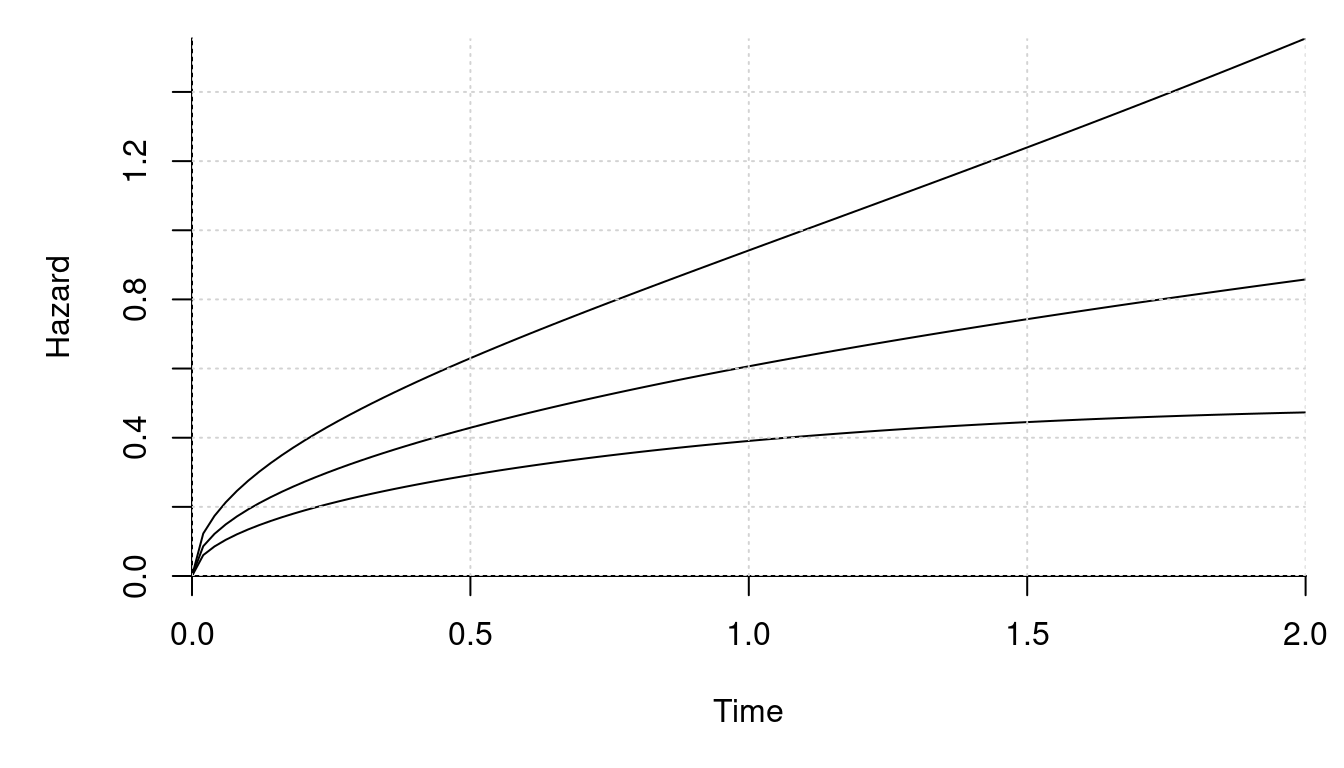# without the markers
plot_surv(
time_fixef = b_term,
time_rng = list(
ns_term(knots = numeric(), Boundary.knots = c(0, 2), intercept = TRUE),
poly_term(degree = 1, raw = TRUE, intercept = TRUE)),
x_range = c(0, 2), vcov_vary = matrix(0, 4, 4), frailty_var = vcov_surv,
ps = c(.25, .5, .75), log_hazard_shift = fixef_surv,
fixef_vary = fixef_vary_surv, associations = associations)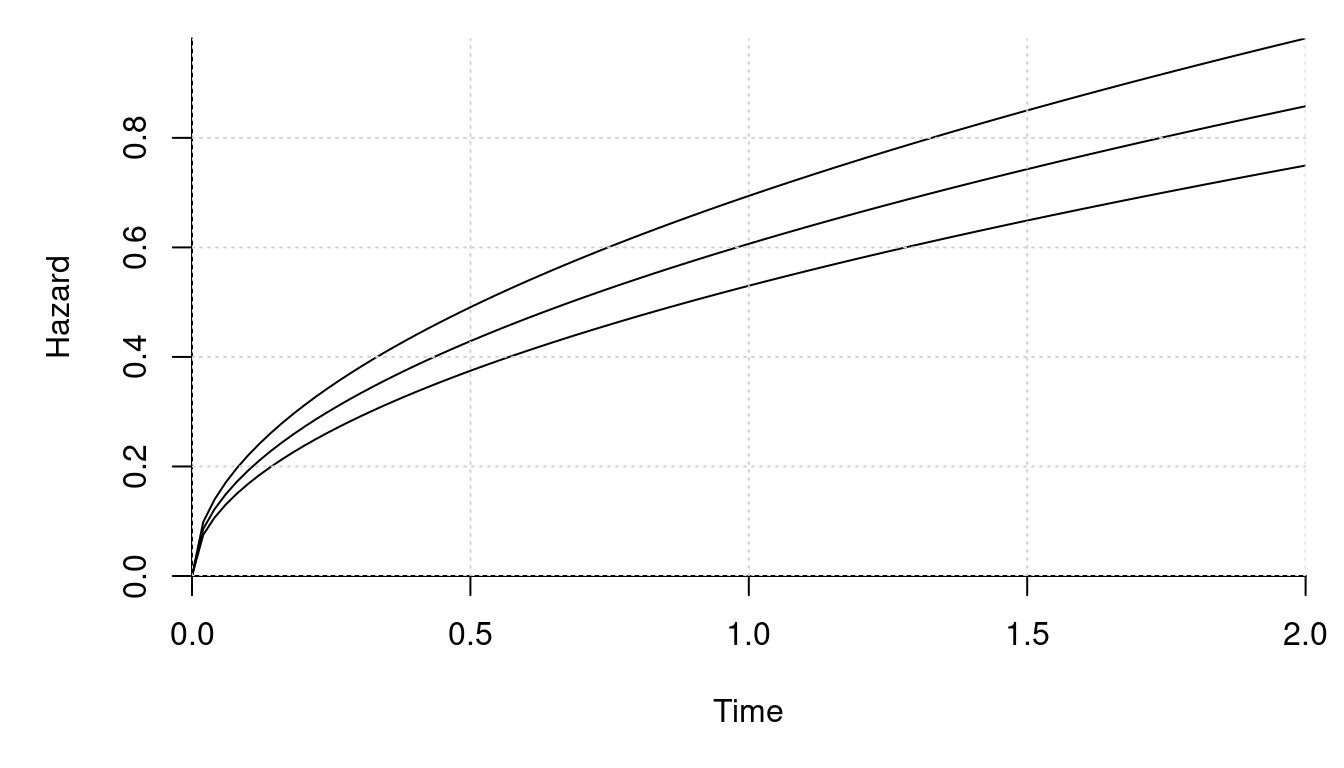# compute a few survival curves and plot them
library(SimSurvNMarker)
# time points where we evaluate the conditional survival functions
tis <- seq(0, 2, length.out = 50)
set.seed(1)
Us <- mvtnorm::rmvnorm(250, sigma = vcov_vary) # the random effects
# compute the conditional survival functions disregarding the fixed effect
surv_vals <- apply(Us, 1, function(U){
expansion <- function(x)
cbind(b_func(x), m_funcs[](x) %*% U[1:2],
m_funcs[](x) %*% U[3:4])

err <- rnorm(1, sd = sqrt(vcov_surv))

eval_surv_base_fun(
ti = tis, omega = c(fixef_vary_surv, associations), b_func = expansion,
gl_dat = get_gl_rule(100), delta = fixef_surv + err)
})

matplot(tis, surv_vals, type = "l", col = gray(0, .1), bty = "l", lty = 1,
ylim = c(0, 1), xaxs = "i", yaxs = "i", xlab = "Time",
ylab = "Conditional survival function")